# Magnetic Island Chains

The analysis of Section 5.16 suggests that a non-zero value of the reconnected magnetic flux at the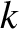th rational surface,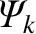, will cause a helical magnetic island chain, with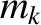periods in the poloidal direction, and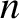periods in the toroidal direction, to open in the immediate vicinity of the surface. Let us investigate the properties of such a chain.

We can write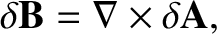(14.71)

where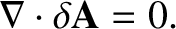(14.72)

Suppose that all terms in the previous equation are of equal importance. It follows from Equation (14.13) that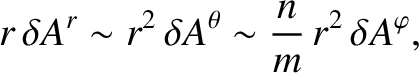(14.73)

and, hence, that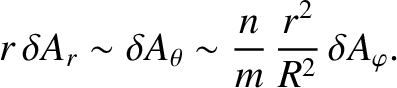(14.74)

Thus, Equations (14.14)–(14.16) and (14.71) yield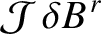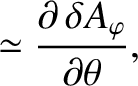(14.75)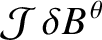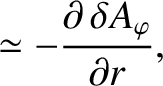(14.76)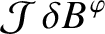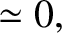(14.77)

where the neglected terms are, at least, of order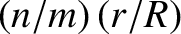smaller than the retained terms. The previous three equations are consistent with Equations (14.44), (14.51), and (14.52) provided that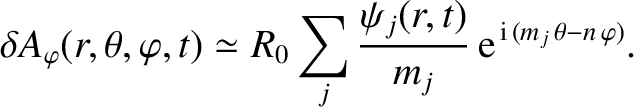(14.78)

Let us search for a function,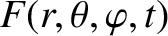, which is such that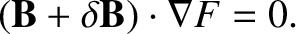(14.79)

It follows from Equations (14.2), (14.4), and (14.6) that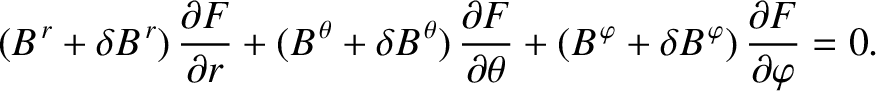(14.80)

Equations (14.3), (14.19)–(14.21), and (14.75)–(14.77) yield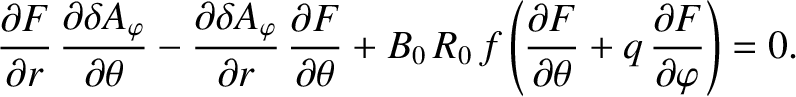(14.81)

Suppose that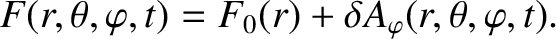(14.82)

The previous two equations give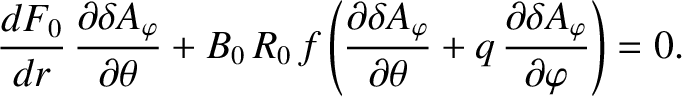(14.83)

According to Equation (14.78), we can write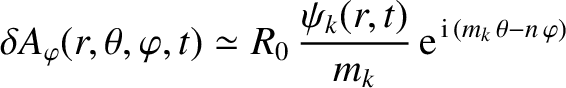(14.84)

in the vicinity of theth rational surface, where we have neglected the non-resonant components of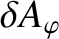(because we do not expect them to open up an island chain at this surface) . The previous two equations yield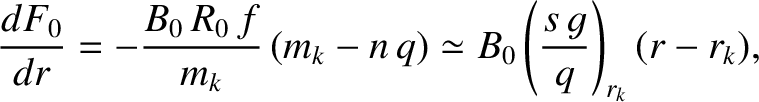(14.85)

where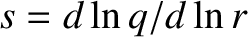is the magnetic shear, and use has been made of Equation (14.18), as well as the fact that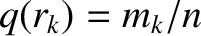. Finally, Equations (14.65), (14.82), (14.84), and (14.85) can be combined to give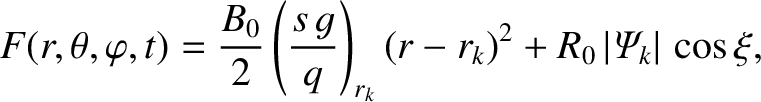(14.86)

where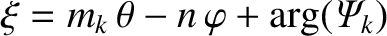. Here, we have taken the (physical) real part of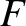, and use has been made of the constant-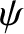approximation that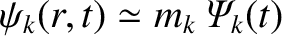in the immediate vicinity of theth rational surface. (See Chapter 5.)

The previous equation can be written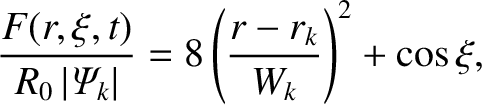(14.87)

where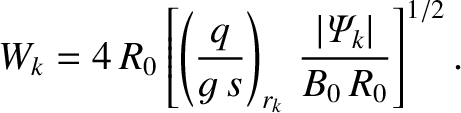(14.88)

Now, according to Equation (14.79), the contours of the function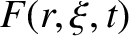map out the perturbed magnetic flux-surfaces in the immediate vicinity of theth rational surface. These contours are shown in Figure 5.7 [with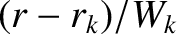playing the role of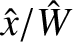]. It can be seen that the reconnected magnetic flux at theth rational surface has indeed opened up a helical magnetic island chain, withperiods in the poloidal direction, andperiods in the toroidal direction, at the surface. Moreover, the full radial width of the island chain (in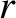) is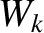. Incidentally, the previous equation is the toroidal generalization of the cylindrical result, (5.129).